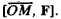# Vector Product

Also found in: Dictionary, Thesaurus, Wikipedia.
Related to Vector Product: vector multiplication

## vector product

[′vek·tər ‚präd·əkt]
(mathematics)

## Vector Product

The vector product of vector a and vector b is the vector denoted by [a, b] and defined as follows: (1) the length of vector [a, b] is equal to the product of the lengths of vectors a and b and the sine of the angle φ between them (the angle between a and b which is less than or equal to π is used); (2) vector [a, b] is perpendicular to both vectors a and b; and (3) the set of three vectors a, b, and [a, b], in accordance with its spatial orientation, always forms either a right-handed or left-handed set. The vector product is widely used in geometry, mechanics, and physics; for example, the moment of a force F, applied to a point M with reference to a point O, is the vector product### REFERENCE

Il’in, V. A., and E. G. Pozniak. Analiticheskaia geometriia. Moscow, 1968.

E. G. POZNIAK

References in periodicals archive ?
It should be noted that the MLFMA is used in each simulation of these examples to accelerate the matrix vector product computations.
Factorization of a dissident map into a vector product and a definite linear endomorphism is no longer possible in general.
In this work, first we defined vector product in [E.
At the same time, the principle of the vectors, especially that of the outer vector product should be maintained.
If the vector product of an odd number of at least three spreading codes at the same spreading factor results in a code of all ones (i.
Because of the linearity of the vector product, the vector IP
The formal structures of the quarks (of the first generation) are presented with outer vector product, its angular movements and the corresponding space types.
Returning to Algorithm 1, we now consider the situation when for the matrix vector product Hs, we approximate the solution of each of the linear systems in steps 2 and 4.
T will play the role of the preconditioner; more precisely, an application of a preconditioner to a given vector x must be equivalent to the matrix vector product Tx.

Site: Follow: Share:
Open / Close